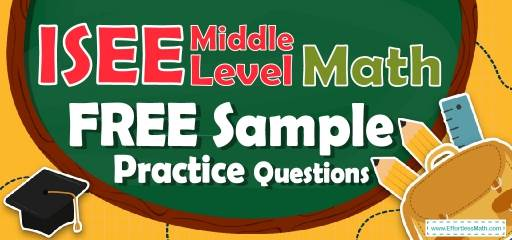# ISEE Middle Level Math FREE Sample Practice QuestionsPreparing your student for the ISEE Middle-Level Math test? To do their best on the ISEE Middle-Level Math test, students need to review and practice real ISEE Middle-Level Math questions.  There’s nothing like working on ISEE Middle-Level Math sample questions to hone their math skills and put them more at ease when taking the ISEE Middle-Level Math test. The sample math questions you’ll find here are brief samples designed to give students the insights they need to be as prepared as possible for the ISEE Middle-Level Math test.

Check out our sample ISEE Middle-Level Math practice questions to find out what areas your student needs to practice more before taking the ISEE Middle-Level Math test!

Help your students start preparing for the 2022 ISEE Middle-Level Math test with our free sample practice questions. Also, make sure to follow some of the related links at the bottom of this post to better understand what kind of mathematics questions students need to practice.

## 10 Sample ISEE Middle Level Math Practice Questions

1- A right cylinder with a radius of 2 inches has volume $$50π$$ cubic inches.

A. The quantity in Column A is greater.

B. The quantity in Column B is greater.

C. The two quantities are equal.

D. The relationship cannot be determined from the information given.

2- $$x$$ is an integer greater than zero.

A. The quantity in Column A is greater.

B. The quantity in Column B is greater.

C. The two quantities are equal.

D. The relationship cannot be determined from the information given.

3-

A. Quantity A is greater.

B. Quantity B is greater.

C. The two quantities are equal.

D. The relationship cannot be determined from the information given.

4-

A. Quantity A is greater.

B. Quantity B is greater.

C. The two quantities are equal.

D. The relationship cannot be determined from the information given.

5- The average of 3, 4, and$$x$$is 3.

A. The quantity in Column A is greater.

B. The quantity in Column B is greater.

C. The two quantities are equal.

D. The relationship cannot be determined from the information given.

6- $$a$$ and $$b$$ are real numbers.

A. The quantity in Column A is greater.

B. The quantity in Column B is greater.

C. The two quantities are equal.

D. The relationship cannot be determined from the information given.

7- $$2x^3+10=64$$
$$120-18y=84$$

A. The quantity in Column A is greater.

B. The quantity in Column B is greater.

C. The two quantities are equal.

D. The relationship cannot be determined from the information given.

8- A swimming pool holds 2,000 cubic feet of water. The swimming pool is 25 feet long and 10 feet wide. How deep is the swimming pool?

A. 2 feet

B. 4 feet

C. 6 feet

D. 8 feet

9- Solve the following equation?
$$6^x=1296$$

A. 3

B. 4

C. 5

D. 6

10- What is the area of the trapezoid?

A. 25

B. 50

C. 100

D. 200

## BestISEE Middle LevelMath Prep Resource for 2022

1- A
The volume of a right cylinder = $$πr^2 h→50π=πr^2 h=π(2)^2 h→h=12.5$$
The height of the cylinder is 12.5 inches which is bigger than 10 inches.

2- D
Choose different values for x and find the value of quantity A.
$$x=1, \space$$then:
Quantity A:
$$\frac{1}{x}+ x= \frac{1}{1}+ 1= 2$$
Quantity B is greater
$$x=0.1,\space then:$$
Quantity A: $$\frac{1}{x} + x= \frac{1}{0.1}+1=10+1=11$$
Quantity A is greater
The relationship cannot be determined from the information given.

3- D
Simply change the fractions to decimals.
$$\frac{4}{5}=0.80$$
$$\frac{6}{7}=0.857…$$
$$\frac{5}{6}=0.8333$$
As you can see, $$x$$ lies between 0.80 and 0.857… and it can be 0.81 or 0.84. The first one is less than 0.833… and the second one is greater than 0.833….
The relationship cannot be determined from the information given.

4- A
Simplify quantity B.
Quantity$$\space B: (\frac{x}{6})^6= \frac{x^6}{6^6}$$
Since the two quantities have the same numerator $$(x^6)$$ and the denominator in quantity B is bigger $$(6^6>6)$$, then the quantity A is greater.

5- C
Quantity A is:
$$\frac{3+4+x}{3}=3→x=2$$
Quantity B is:
$$\frac{2+(2-6)+(2+4)+(2×2)}{4}=2$$

6- C
Choose different values for a and b and find the values of quantity A and quantity B.
$$a=2 \space and \space b=3, \space then:$$
Quantity A: $$|2-3|=|-1|=1$$
Quantity B: $$|3-2|=|1|=1$$
The two quantities are equal.
$$a=-3 \space and \space b=2, \space$$ then:
Quantity A: $$|-3-2|=|-5|=5$$
Quantity B: $$|2-(-3)|=|2+3|=5$$
The two quantities are equal.
Any other values of a and b provide the same answer.

7- A
$$2x^3+10=64→2x^3=64-10=54→x^3=\frac{54}{2}=27$$
$$→x=\sqrt{27}= \sqrt{3^3}= 3$$
$$120-18y=84→-18y=84-120=-36→y=\frac{-36}{-18}=2$$

8- D
Use formula of rectangle prism volume.
$$V = (length) (width) (height)$$
$$⇒ 2000 = (25) (10) (height)$$
$$⇒ height = 2000 ÷ 250 = 8$$

9- B
$$1269=6^4 →6^x=6^4→x=4$$

10- B
The area of trapezoid is: $$\frac{9 + 11}{2} ×5=50$$

## The Best Books to Ace the ISEE Middle Level Math Test

### What people say about "ISEE Middle Level Math FREE Sample Practice Questions - Effortless Math: We Help Students Learn to LOVE Mathematics"?

No one replied yet.

X
23% OFF

Limited time only!

Save Over 23%

SAVE $5 It was$21.99 now it is \$16.99